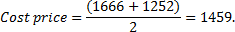## Profit and Loss For SBI PO Set – 5

1) Shalini and Nalini invested Rs. 80,000 each and started a business. After one year Shalini invests an additional amount of Rs. 20,000 and Nalini withdraws Rs. 30,000. At the end of two years they earn a profit of Rs. 93,000. What will be Nalini’s share in the profit?

a) Rs. 39,000

b) Rs. 54,000

c) Rs. 52,000

d) Rs. 36,000

e) None of these

a) Rs. 39,000

Explanation

Shalini share: Nalini share

80000+(80000+20000):80000+(80000-30000)
.180000:130000

18:13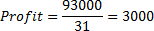Nalini share=3000×13=Rs.39000

2) A shopkeeper sold a watch for Rs. 5076 at the loss of 6%. What is the cost price of the watch?

a) Rs. 5,200

b) Rs. 5,600

c) Rs. 5,400

d) Rs. 4,752

e) None of these

c) Rs. 5,400

Explanation

Selling price 94%=5076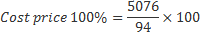=Rs.5400

3) A and B jointly invest Rs. 2100 and Rs. 3100 respectively in a F. A is an active partner and he gets 25% of the profit separately. If their business yiels them total Rs. 1040 as profit what will be the gain of each of them?

a) Rs. 415, Rs. 625

b) Rs. 575, Rs. 465

c) Rs. 515, Rs. 525

d) Rs. 560, Rs. 480

d) None of these

b) Rs. 575, Rs. 465

Explanation

Total profit is 1040,

out of which A gets 25% separately, So, 25% 1040 = 260
.B gets (1040 – 260) = 780

Distributed among both in ratio 2100 : 3100 or 21 : 31
.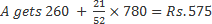B gets 31/52 ×780=Rs.465

4) Manhar sold an item for Rs. 8,400 and incurred a loss of 25%. At what price should he have sold the item to have gained a profit of 40%?

a) Rs. 15,680

b) Rs. 16,220

c) Rs. 14,540

d) Cannot be determinedc

e) None of these

a) Rs. 15,680

Explanation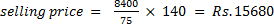5)  The owner of a furniture shop charges his customers 15% more than the cost price. If a customer paid Rs. 9,039/- for a sofa set, then what is the cost price of the sofa set?

a) Rs. 7,680

b) Rs. 7,860

c) Rs. 7,880

d) Rs. 7,660

e) None of these

b) Rs. 7,860

Explanation

Selling price=115% of cost price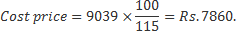[/su_spoiler]

6) The cost price of an article is Rs. 390. If it is to be sold at a profit of 3.12 percent, how much would be its approximate selling price?

a) Rs. 410

b) Rs. 402

c) Rs. 417

d) Rs. 420

e) Rs. 442

b) Rs. 402

Explanation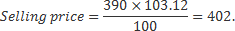7) Suresh sold an item for Rs. 5,625 and incurred a loss of 25%. At what price should he have sold the item to have gained a profit of 25%?

a) Rs. 9,375

b) Rs. 10,500

c) Rs. 8,250

d) Cannot be determined

e) None of these

a) Rs. 9,375

8) Sumit purchased an item for Rs. 6,500 and sold it at the gain of 24%. From that amount he purchased another item and sold it at the loss of 20%. What is his overall gain/loss?

a) Loss of Rs. 42

b) Gain of Rs. 42

c) Loss of Rs. 52

d) Neither gain nor loss

e) None of these

c) Loss of Rs. 52

Explanation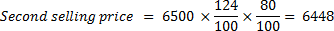.Loss = 6500 – 6448 = Rs. 52

9) The profit earned after selling a T-shirt for Rs. 575 is the same as loss incurred after selling the same T-shirt for Rs. 295. What is the cost price of the T-shirt?

a) Rs. 425

b) Rs. 445

c) Rs. 430

d) Rs. 450

e) None of these

e) None of these

Explanation:

Cost price=x

Then, 575-x=x-295

x=435.

10) The profit earned after selling on article for Rs. 1666 is the same as the loss incurred after selling the article for Rs. 1252. What is the cost price of the article? 1564

a) 564

b) 1574

c) 1504

d) 1503

e) None of these

e) None of these

Explanation